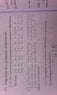# Square of determinant is symmetric

• I
• debjit625

#### debjit625

This property is given in my book.
The square of any determinant is a symmetric determinant.
Well it works when I take a determinant say 3x3 and multiply it by itself using row to row multiplication.
But it fails if I multiply using row to column.

Thanks

What is a symmetric determinate? In which sense is it symmetric? In the end it is a single value.

a symmetric matrix is just a matrix that is the same if transposed. if you multiply a matrix by its transpose you will always get a symmetric matrix as a result. this could be what your section on matrixes means.

so here we go working with matrixes. my i hope you have a great day.

Thanks fresh_42 and akeleti8
Guys I have the idea what u people are saying but have a look ,of the pic I uploaded its from the book

Thanks

#### Attachments

•IMG_20160525_124745.jpg
22.2 KB · Views: 1,060
What is the name of the book?
It is not using terms correctly.
I think it means "matrix" where it writes "determinant".
It could be that they are interested in eventually taking the determinant of a matrix.

What is the name of the book?
It is not using terms correctly.
I think it means "matrix" where it writes "determinant".
It could be that they are interested in eventually taking the determinant of a matrix.
That was what I thought. Then I took a closer look on its squared matrix. The entry ##(i,j)## of ##A^2## is the product, notated by an ##\times## of the ##j-##th row with the ##i-##th row. A strange notation and usage of the transposed matrix. One has to decode it first.

Edit: So @akeleti8's explanation on the product of a matrix with its transposed is the answer here, only that the book uses a strange way to write and name it.
##A^τ \cdot A## is symmetric because ##(A^τ \cdot A)^τ = A^τ \cdot (A^τ)^τ = A^τ \cdot A##
It seems the author calls a determinate symmetric, if it is the determinate of a symmetric matrix.
Is it a school book?

Last edited:
No its a standard college text.there are more books which have this statement just Google the property u would find some .

No its a standard college text.there are more books which have this statement just Google the property u would find some .
The book might be standard, the notation is not. I just googled "1 bigger than 2" and got more than 107,000,000 hits.
Matrix multiplication is row times column and nothing else. I don't want to find crude new notations. A transposed matrix should be noted as such. The author simply uses of the fact that ##\det(A) = \det(A^τ)## and thus by putting ##\det##, here notated by ##|\,.|##, around the matrices he is allowed to arbitrarily jump between ##\det(A)## and ##\det(A^τ)## or using ##|A| \times |B| = |B| \times |A|## without need or explanation. This confuses the reader without need or any advantage. In addition "symmetric determinate" for "determinate of a symmetric matrix" is more than sloppy. A symmetric real number makes no sense (25,500,000 hits - starting with matrices!).

Edit: The equation in the book goes: ##|A|^2 = |A \cdot A| = |A| \cdot |A| = |A| \cdot |A^τ|## by mentioning that ##A \cdot A^τ## is symmetric. The steps, however, are not mentioned - at least not on the page you showed us.

Last edited:
search for "The square of any determinant is a symmetric determinant."
its determinant not determinate(I assume by determinate you mean just the real value).And all those thing you are saying I totally agree .
I am 1st year student of physics honors,may be not a lot but I know a bit about linear algebra and I understand what you are trying to say. But my question is do anybody have any idea what it is? I myself is confused ,because its not only in my book but some other books are too showing this ...

Here are some books which you can find in google books review
A Textbook of Matrices by Hari Kishan.
A Treatise on the Theory of Determinants

By Thomas Muir, William Henry Metzler

@robphy
"I think it means "matrix" where it writes "determinant"."
No we
have separate chapter for matrix.

Thanks